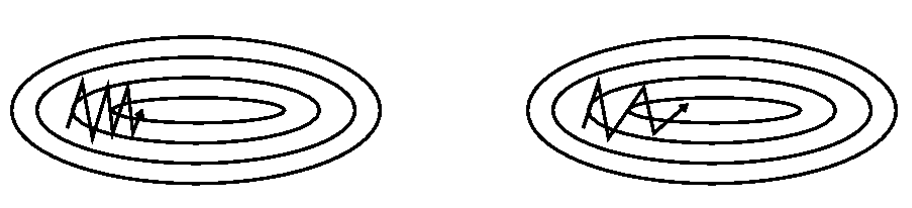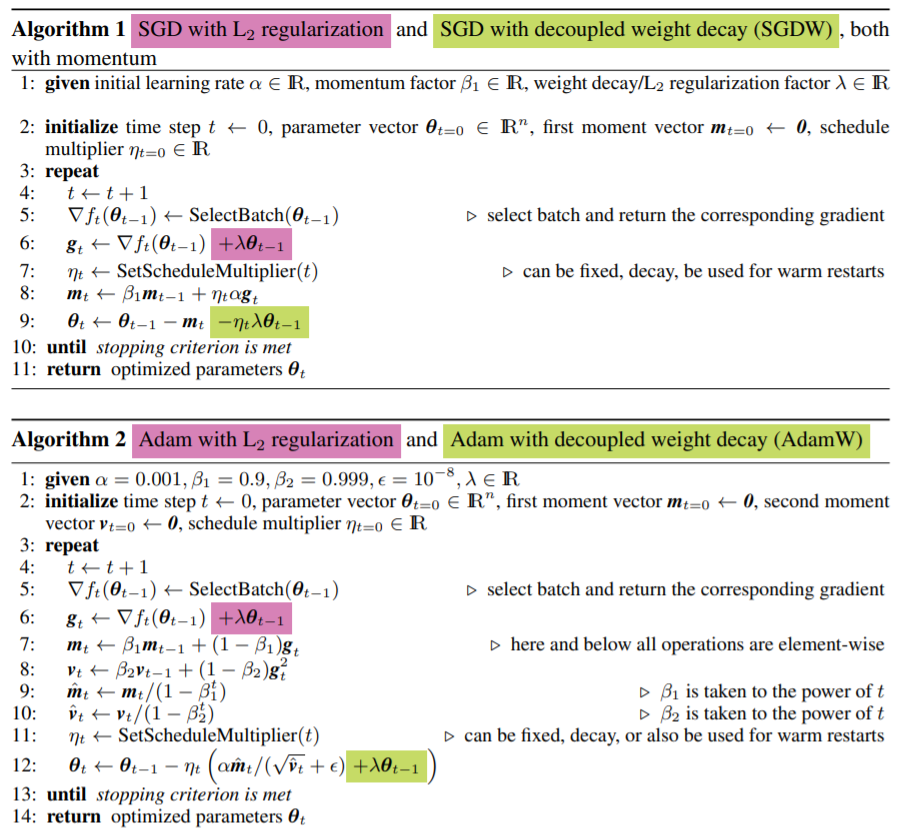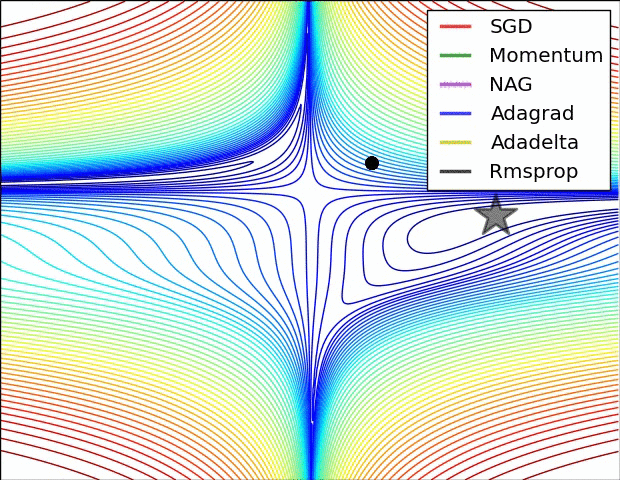# 深度学习优化器

## ♠ 初级的优化器

$\theta = \theta - \eta \cdot \nabla_{\theta} J(\theta)$

for i in range(epochs):
params = params - lr * grad


• 优点：如果是凸优化一定能取得全局最优解，如果是非凸优化可以取得局部最优解。
• 缺点：如果数据量过大会使得优化时间变长，优化变得十分缓慢，而且对 memory 也有一定要求。

$\theta = \theta - \eta \cdot \nabla_{\theta}J(\theta;x^{(i)};y^{(i)})$

SGD 可以避免 BGD 因为大数据集而造成的冗余计算，比如 BGD 会对相似的数据进行重复计算。SGD 则是每次只选择一个样本的数据来进行更新梯度，伪代码如下：

for i in range(epochs):
random_shuffle(dataset)
for data_i in dataset:
params = params - lr * grad


• 优点：
• 由于一次只用一个数据，因此梯度更新很快
• 当然也可以进行在线学习（不用收齐所有数据）
• 也会处于一个高 variance 的状态，更新时 loss 比较震荡，可能会使得其跳出局部最优点到达一个更好的局部最优。
• 缺点：
• 也正因为震荡，很难收敛于一个精准的极小值。但实验表明，只要对学习率进行调整就可以使得 SGD 的最终收敛效果与 BGD 一致。

$\theta = \theta - \eta \cdot \nabla_{\theta}J(\theta;x^{(i:i+n)};y^{(i:i+n)})$

for i in range(epochs):
random_shuffle(dataset)
for batch_data in dataset:
params = params - lr * grad


• 选择合适的学习率仍然是一个玄学
• 学习率 schedule 需要预设不能自适应数据集的特点
• 学习率针对所有参数，而并非所有参数需要同样的学习率
• 对于非凸问题极易陷入局部最优

## ♣ 进阶的优化器

### MomentumSGD 是每次选择一个数据进行梯度更新，在”峡谷“地带时，梯度经常异号就 loss 反复震荡（如上图）。这好比这辆车每开一步就换了一个司机（数据产生的梯度），每个司机的想法都不同。特别是到局部最优的时候，由于梯度始终为 0 使得每个司机上车后都说刹车别走。

\begin{aligned} v_t &= \gamma v_{t-1} + \eta \nabla_{\theta} J(\theta_{t-1}) \\ \theta_t &= \theta_{t-1} - v_t \end{aligned}

Momentum 虽然会使考虑之前梯度与当前梯度来决定当前权重如何更新，但是它还不够聪明，它不会预测未来可能的情况。由上面最后一式我们可以知道权重是由动量项更新的 $$v_t$$ 来进行更新。我们来看看 NAG 是如何做的：

\begin{aligned} v_t &= \gamma v_{t-1} + \eta \nabla_{\theta} J(\theta_{t-1} - \gamma v_{t - 1}) \\ \theta_t &= \theta_{t-1} - v_t \end{aligned}

## ♥ 智能的优化器

\begin{aligned} v_t &= \beta v_{t-1} + (1 - \beta)\theta_{t} \\ v_{t-1} &= \beta v_{t-2} + (1 - \beta)\theta_{t - 1} \\ &\dots \\ v_{1} &= \beta v_{0} + (1 - \beta)\theta_{1} \end{aligned}

$v_t = (1 - \beta)\beta\theta_{t - 1} + (1 - \beta)\beta^2\theta_{t - 2} + \cdots + (1 - \beta)\beta^{n - 1}\theta_{1}$

$$\beta$$ 一般是一个小于 1 的数，由上式可知 t 时刻的变量会由前 t - 1 个时刻的变量共同决定，但每个变量前加了一个关于 $$\beta$$ 的指数级系数，离 t 时刻越远的权重越小，若是 $$\beta =0.9$$ 第 20 个数的权重都在 0.01 左右了，后面的影响会更小。指数平均加权由此而来。其实我们很容易发现：

$v_{1} = (1 - \beta)\theta_{1}$

$\hat{v}_{t} = \frac{v_{t}}{1 - \beta^t}$

$g_{t,i} = \nabla_{\theta_t}J(\theta_{t, i})$

$$g_{t,i}$$ 代表了第 t 步的第 i 个参数 $$\theta_{t, i}$$ 的梯度，梯度更新则使用下列式子：

$\theta_{t + 1, i} = \theta_{t, i} - \frac{\eta}{\sqrt{G_{t,ii}+\epsilon}} \cdot g_{t,i} \tag{a*}$

$$G_t \in \mathbb{R}^{d \times d}$$ 是一个对角矩阵，对角线上的元素 $$G_{t,ii}$$ 计算如下：

$G_{t,ii} = G_{t - 1,ii} + g_{t, i}^2 = \sum_{k = 0}^t g_{k, i}^2$

$\theta_{t} = \theta_{t - 1} - \frac{\eta}{\sqrt{G_{t - 1}+\epsilon}} \odot g_{t - 1}$

$E[g^2]_t = \gamma E[g^2]_{t-1} + (1 - \gamma)g^2_t$

$\Delta \theta_t = -\frac{\eta}{\sqrt{E[g^2]_t + \epsilon}}g_t = -\frac{\eta}{RMS[g]_t}g_t$

\begin{aligned} E[\Delta\theta^2]_t &= \gamma E[\Delta\theta^2]_{t-1} + (1 - \gamma)\Delta\theta^2_t \\ RMS[\Delta\theta]_t &= \sqrt{E[\Delta\theta^2]_t + \epsilon} \end{aligned}

$x_n = x_{n-1} - \frac{f^{\prime}(x_{n-1})}{f^{\prime \prime}(x_{n-1})}$

\begin{aligned} \because \quad&\Delta \theta \approx \frac{J^{\prime}(\theta)}{J^{\prime \prime}(\theta)} \\ \therefore \quad&\frac{1}{J^{\prime \prime}(\theta)} = \frac{\Delta \theta_t}{J^{\prime}(\theta)} \approx -\frac{RMS[\Delta\theta]_{t-1}}{RMS[g]_{t}} \end{aligned}

$\theta_t = \theta_{t-1} - \frac{RMS[\Delta\theta]_{t-2}}{RMS[g]_{t-1}}g_{t-1}$

$\theta_t = \theta_{t-1} - \frac{\eta}{RMS[g]_{t-1}}g_{t-1}$

Adaptive Moment Estimation 有点像是 Momentum 和 RMSProp 的结合体，首先对梯度，梯度的平方使用指数加权平均：

\begin{aligned} m_t = \beta_1 m_{t-1} + (1 - \beta_1)g_t \\ v_t = \beta_2 v_{t-1} + (1 - \beta_2)g_t^2 \end{aligned}

\begin{aligned} \hat{m}_t = \frac{m_{t}}{1 - \beta_1^t} \\ \hat{v}_t = \frac{v_{t}}{1 - \beta_2^t} \end{aligned}

$\theta_t = \theta_{t-1} - \frac{\eta}{\sqrt{\hat{v}_{t-1}}+\epsilon}\hat{m}_{t-1}$AdamW 想做的最主要是认为 Adam 没有正确使用进行权重衰减。当我们使用 L2 正则化时，会在 loss 后面添加一个权重的平方项： $$loss = loss_{orig}(\theta) + \frac{1}{2}\lambda\theta^2$$ 添加这个平方项的目的，是对其求导有一个权重衰减的效果，比如我们如果使用的 SGD 则权重更新的表达式为（最后一项是权重衰减）： $$\theta_t = \theta_{t-1} - \eta \cdot \nabla J(\theta_{t-1}) - \eta\lambda \theta_{t-1}$$ 如上图所示，当我们使用动量的时候，对 loss 再添加正则化项，然后求导获得权重衰减。其效果不再与 SGD 中的相同，因为动量会累积之前的梯度，因此当使用动量的时候，权重更新的公式为：

$m_t = \beta m_{t-1} + \eta (1-\beta)\nabla_{\theta} J(\theta_{t-1}) + \eta \lambda (1-\beta)\theta_{t-1}\\ \theta_t = \theta_{t-1} - m_t - \eta \lambda (1-\beta)\theta_{t-1}$

$\theta_t = \theta_{t-1} - \frac{\eta}{\sqrt{\hat{v}_{t-1}}+\epsilon}\hat{m}_{t-1} - \eta \lambda \theta_{t-1}$posted @ 2021-06-30 20:58  FromL77  阅读(3235)  评论(0编辑  收藏  举报